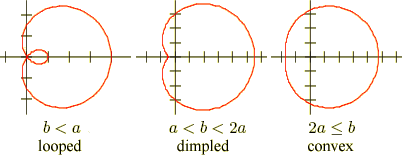index: click on a letter A B C D E F G H I J K L M N O P Q R S T U V W X Y Z A to Z index index: subject areas numbers & symbols sets, logic, proofs geometry algebra trigonometry advanced algebra & pre-calculus calculus advanced topics probability & statistics real world applications multimedia entrieswww.mathwords.com about mathwords website feedback

 Limaçon A famliy of related curves usually expressed in polar coordinates. The cardioid is a special kind of limaçon.   Limaçon:   r = b + a cos θ (horizontal, pictured below)  or  r = b + a sin θ (vertical)Note: If a = b the curve is a cardioid.   See also